Levi John Wolf¶

Lecturer in Quantiative Human Geography, Center for Multilevel Modeling, University of Bristol

Visiting Fellow, Center for Spatial Data Science, University of Chicago

@levijohnwolf

With Gill v. Whitford being argued at the Supreme Court, it might make a little sense to try and distill a few concepts behind how these measures actually get calculated. This is a really short take from the work I've conducted in my doctoral dissertation, Spatializing Partisan Gerrymandering Forensics. Below, I'll try to explain basics in the estimation of partisan bias from observed elections. I'll show two simple empirical measures, the Median-Mean discrepancy & Efficiency Gap, as well as two counterfactual measures, the bonus-at-median and observed bonus. This'll mix a little math, a little Python, and some reading, so it is hopefully helpful for people who dabble in any of those.

My main concern with this is to provide an illustration about where the "gobbledegook" seeps into the process of estimating and understanding partisan bias. I hope to do this in a few blogposts. This first one just deals with the mechanics of how a partisan bias measure is typically calculated and presented. I say "typically," but in earnest, there's a wide variety of methods & approaches here. I'm showing only one possible one. That itself is a point of gobbledegook!

Second, I hope to show that it's not efficiency gap or bust. The efficiency gap is not new in its suggestion of providing a single statistic of how biased an electoral system is. In fact, a very similar idea to the efficiency gap was examined as far back as 1959. I'll present a few measures that are used now & might be used in the future. I wont talk about the jurisprudence, since I'm neither an expert nor am I confident in how the Whitford case will turn out, especially with respect to targeting first amendment claims in constitiutional jurisprudence. Regardless of the Supreme Court, my research leads me to believe that state-specific reforms are likely the single best place to tackle partisan gerrymandering.

This jupyter notebook is partially funded by NSF Grant #1657689. (I never thought I'd disclose a jupyter notebook...)

Structure of Bias Measures¶

There are a ton of partisan bias measures, but they tend to fall into two types.

1. Counterfactual: These measures are designed to be computed at a given electoral configuration. This might be when parties recieve the same level of vote or when one party wins the smallest possible majority. Typically, these "reference scenarios" are constructed by first estimating a model of elections and then simulating many elections under the required conditions. The measures, when applied to the simulated situation, then provide an estimate of bias.
2. Empirical: These measures are designed to be computed directly from observed election results. They do not require any statistical model for vote shares, but they can be adapted to use the output from electoral model.

Conceptually, the two are quite distinct. Since the counterfactual measures require the evaluation of a specific electoral scenario, the bias measurement summarizes our belief about what would happen were that condition to arise. Critically, this means that the counterfactual measures do not necessarily summarize the electoral system as expeirenced. Further, since empirical measures can be applied to simulated elections too, their sensitivity can be examined by simulating elections close to (but not exactly at) the observed electoral conditions.

I'll talk a little about the empirical measures first, to show how they work. I'll do this example using the data I built for my dissertation. I've got the Congressional elections in Illinois for 2014 and 2016 on hand for this illustration.

In :
import geopandas as gpd
import pandas as pd
import numpy as np
import seaborn.apionly as sns
import matplotlib.pyplot as plt
sns.set_context("notebook", font_scale=1.75)
%matplotlib inline

Empirical Measures¶

Like I said above, these tend to take the observed election results & cross-tabulate them in some novel way. One of the simplest you could use (stated by McDonald & Best (2016)) is the difference between the median and mean district vote share. Here, I'll just focus on using the Democratic vote share of the two-party vote. In district $i$, two-party Democratic vote share $h_i$ is simply the votes cast for Democrats $d_i$ divided by the vote cast for Democrats and Republicans ($r_i$):

$$h_i = \frac{d_i}{d_i + r_i}$$

This is a simplification to remove minor third parties, and works well in most modern congressional elections.

The last thing to make sure to distinguish is that the party average vote share is not the same as the statewide vote share. That is:

$$\frac{\sum_i h_i}{N} \neq \frac{\sum_i d_i}{\sum_i d_i + r_i}$$

In fact, the "statewide" Democratic vote, being the fraction of all votes cast in the state for Democrats, is the weighted average of $h_i$ using turnout $m_i$ as the weights.

Median vs. Mean¶

Accounting for this, McDonald & Best suggest the difference between the median vote share and the average district vote share represents a measure of the advantage a party experiences. Computed for Illinois in 2014 and 2016:

In :
f,ax = plt.subplots(1,2, figsize=(4.2*3, 3), sharex=True, sharey=True)
for i,(year, chunk) in enumerate(data.groupby('year')):
sns.kdeplot(chunk.vote_share.values, ax=ax[i])
ax[i].vlines(chunk.vote_share.mean(),0,.25, linestyle='--', label='mean')
ax[i].vlines(chunk.vote_share.median(),0,.25, linestyle=':', label='median')
ax[i].set_ylabel(year)
ax[i].set_xlabel("Two-Party Vote %D")
ax[i].set_xlim(0,1)
ax.legend(frameon=False, loc='upper right')
Out:
<matplotlib.legend.Legend at 0x7f13bd68b9b0>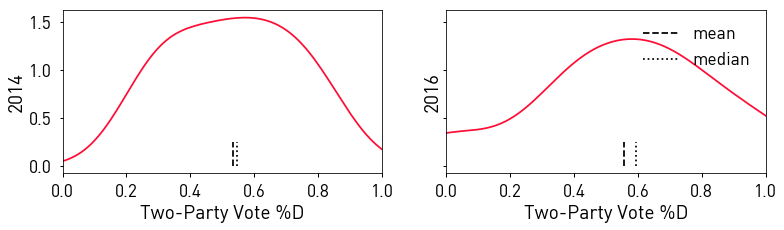In :
data.groupby('year').vote_share.apply(lambda x: x.median() - x.mean())
Out:
year
2014    0.011747
2016    0.037907
Name: vote_share, dtype: float64

So, this means that the median Democratic district had slightly higher Democratic two-party vote share than the mean district's vote share by about 1% in 2014 and by about 4% in 2016.

By McDonald & Best's argument, the median represents the observed vote share required to win exactly half of the seats in the state. If it's harder for Democrats to win half of the seats in the state (i.e. Democrats require a larger vote share in each district) than the Democrats win on average, they experience a malus. The size of this penalty is the difference between the median and the mean, and it reflects the "extra" percentage vote the Dems need or do not need to win a majority in the state.

Efficiency Gap¶

The efficiency gap (McGhee (2014), also McGhee & Stephanopolous (2015)) is the latest in a long line of what I'd call "contest size" measures. The first I'm aware of in this line date back to Brooks (1960), "The analysis of distorted representation in two-party, single-member elections," Political Science, 12, 158-167 (See also a dissertation in 2010 on the topic). Conceptually, these measures have aimed to explore whether or not parties "waste" an equal number of votes.

Conceptualized another way, the measures aim to examine whether the "cost" of a seat is consistent. If one party tends to lose big contests and not win many smaller contests, then the total number of votes cast for that party will be much larger than their anticipated share of the Congressional delegation (or legislature). Thus, the efficiency gap is one new method of expressing this concept.

McGhee (2014) considers votes "wasted" in two ways. I'd call these surplus votes, the "extra" votes cast for a candidate who wins above %50, and lost votes, those cast for losing candidates. Since surplus votes do not affect the winner, they might be shifted to another district to improve their party's position. Likewise, lost votes do not affect the winner, so they might be shifted to another district, too. In either case, the party's "efficiency" would increase, in terms of the number of seats won per votes cast.

Hence, when parties have a large difference in efficiency, the efficiency gap characterizes this waste differential. Letting waste for Republicans be $W_R$ and waste for Democrats be $W_D$, the efficiency gap is the difference in waste divided by the total number of votes cast:

$$\frac{W_R - W_D}{\sum_i d_i + r_i}$$

I won't go into formalizing the computation of $W_R$, but consult McGhee (2014) or my dissertation for further details. Below, I'll compute it, in Python:

In :
egap = dict()
for year, chunk in data.groupby('year'):
Rwin = chunk.query('vote_share < .5')
Dwin = chunk.query('vote_share >= .5')

Rsurplus = ((1-Rwin.vote_share) -.5) * Rwin.turnout
Rlost = ((1 - Dwin.vote_share)) * Dwin.turnout

Dsurplus = (Dwin.vote_share - .5) * Dwin.turnout
Dlost = (Rwin.vote_share) * Rwin.turnout

Dwaste = Dsurplus.sum() + Dlost.sum()
Rwaste = Rsurplus.sum() + Rlost.sum()

egap_t = (Rwaste - Dwaste) / chunk.turnout.sum()
egap.update({year:egap_t})
pd.Series(egap)
Out:
2014   -0.019956
2016    0.002011
dtype: float64

So, this means that, in 2014, the Republicans had slightly lower waste then Democrats, and thus experienced an advantage of around 1%. Then, in 2016, Democrats wasted slightly fewer votes than Republicans, experiencing a bonus of .2% (yes, that's nearly zero... a fraction of a percent).

There's also a "simplified form" of the efficiency gap, but I don't think it's even worth discussing this. The simplified version assumes that turnout is equal over districts. But, if you have the data, why assume this? So, I won't consider it here.

I acknowledge that sometimes, you won't have turnout information, and in that case it may be appropriate to assume the districts are equal, so this isn't a general prohibition.

Uncontested Elections¶

An astute reader might wonder what happens when a district isn't contested. This is a great question, since there are usually quite a few uncontested elections in each year. In Illinois:

In :
data.query('vote_share == 0 or vote_share == 1')
Out:
vote_share turnout inc year congress statedist state_name dem_votes geometry
2 1.0 225320.0 1 2016 115 1703 illinois 225320 POLYGON ((-88.141966 41.548491, -88.141936 41....
3 1.0 171297.0 1 2016 115 1704 illinois 171297 POLYGON ((-87.920632 41.886324, -87.920631 41....
13 0.0 274554.0 -1 2016 115 1715 illinois 0 POLYGON ((-90.038111 38.670688, -90.03807 38.6...
16 0.0 259722.0 -1 2016 115 1716 illinois 0 POLYGON ((-89.868379 41.149183, -89.8683659999...

There were 4, all in 2016. There were 2 Democratic uncontested districts (3,4) and two Republican (15,16). A reasonable question might be to ask how the gap (or any measure) is different if those districts were to have been contested. There are two ways to do this:

Contested-Only¶

Just compute the statistics for the elections that are contested:

In :
egap_contested = dict()
for year, chunk in data.query('vote_share < 1 and vote_share > 0').groupby('year'):
Rwin = chunk.query('vote_share < .5')
Dwin = chunk.query('vote_share >= .5')

Rsurplus = ((1-Rwin.vote_share) -.5) * Rwin.turnout
Rlost = ((1 - Dwin.vote_share)) * Dwin.turnout

Dsurplus = (Dwin.vote_share - .5) * Dwin.turnout
Dlost = (Rwin.vote_share) * Rwin.turnout

Dwaste = Dsurplus.sum() + Dlost.sum()
Rwaste = Rsurplus.sum() + Rlost.sum()

egap_t = (Rwaste - Dwaste) / chunk.turnout.sum()
egap_contested.update({year:egap_t})
pd.Series(egap_contested)
Out:
2014   -0.019956
2016   -0.013644
dtype: float64

In this case, removing the uncontested districts in 2016 causes the sign of the efficiency gap to flip! and it looks more like the gap from 2014!

This should illustrate that the statistics are often quite sensitive to these far-out uncontested districts. How to account for them should always be at the forefront of your mind when discussing advantage.

Imputation¶

Another way would be to examine the anticipated Democratic vote share in the uncontested districts if they were to have been contested. One way we can do this is using the 2014 vote shares. To make sure we're aligning things properly, I'll do a self-merge on the district number:

In :
stacked = data.query("year == 2014").merge(data.query('year == 2016')[['vote_share','statedist', 'inc', 'turnout']],
on='statedist', suffixes=('','_2016'))

And, we see that last year's vote share is usually a pretty good predictor of this year's vote share:

In :
f = plt.figure(figsize=(5,5))
sns.regplot('vote_share',
'vote_share_2016', data=stacked, ci=99)
plt.xlabel("TPV %D, 2014")
plt.ylabel("TPV %D, 2016")
Out:
<matplotlib.text.Text at 0x7f13bd7ea0b8>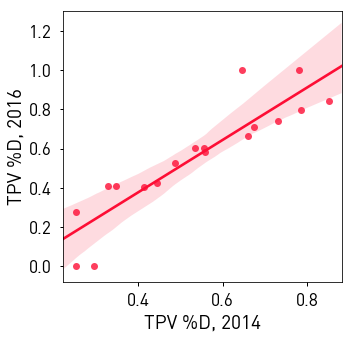So, one strategy is to use the relationship between last year's vote share and this year's vote share (plus any other info we have on hand) and predict the uncontested election's vote shares in 2016 as out-of-sample predictions.

Here, I'll just use the simplest possible model, a linear regression of 2016 onto 2014's two-party vote shares. I'll also use the ordinal information about the incumbency in a district. There are other more sophisticated models we could use, but you'll find that fit is rather good for these "two-cycle" models:

In :
import statsmodels.formula.api as smf
In :
model = smf.ols('vote_share_2016 ~ vote_share + inc_2016',
data=stacked.query('vote_share_2016 < 1 & vote_share_2016 > 0')).fit()
In :
model.summary()
/home/ljw/anaconda3/envs/ana/lib/python3.6/site-packages/scipy/stats/stats.py:1334: UserWarning: kurtosistest only valid for n>=20 ... continuing anyway, n=14
"anyway, n=%i" % int(n))
Out:
Dep. Variable: R-squared: vote_share_2016 0.980 OLS 0.976 Least Squares 265.8 Wed, 04 Oct 2017 4.87e-10 15:06:24 32.740 14 -59.48 11 -57.56 2 nonrobust
coef std err t P>|t| [0.025 0.975] 0.1346 0.042 3.235 0.008 0.043 0.226 0.7979 0.077 10.378 0.000 0.629 0.967 0.0289 0.014 2.088 0.061 -0.002 0.059
 Omnibus: Durbin-Watson: 0.729 1.441 0.695 0.619 -0.072 0.734 1.98 14.6

So, in 14 observations, we obtain an $R^2$ in the high 90s. This is chiefly driven by the 2014 vote share.

With this info, we can predict the vote shares out of sample.

First, I'll select out only the uncontested districts:

In :
uncs = stacked.query('vote_share_2016 == 0 or vote_share_2016 == 1')
not_unc = stacked.index.difference(uncs.index)

Then, using statsmodels's predict method, I'll predict the vote shares that we would've observed had these districts been contested given what we saw in Illinois in 2016.

In :
uncs['imputed'] = model.predict(uncs[['vote_share', 'inc_2016']])
/home/ljw/anaconda3/envs/ana/lib/python3.6/site-packages/ipykernel_launcher.py:1: SettingWithCopyWarning:
A value is trying to be set on a copy of a slice from a DataFrame.
Try using .loc[row_indexer,col_indexer] = value instead

See the caveats in the documentation: http://pandas.pydata.org/pandas-docs/stable/indexing.html#indexing-view-versus-copy
"""Entry point for launching an IPython kernel.

I've gotta do some further chicanery to get the results all collated together:

In :
stacked.loc[uncs.index, 'imputed'] = uncs.imputed
stacked.loc[not_unc, 'imputed'] = stacked.loc[not_unc, 'vote_share_2016']

And now, our imputed vote shares:

In :
sns.regplot(stacked.vote_share_2016, stacked.imputed)
plt.xlim(0,1)
plt.ylim(0,1)
Out:
(0, 1)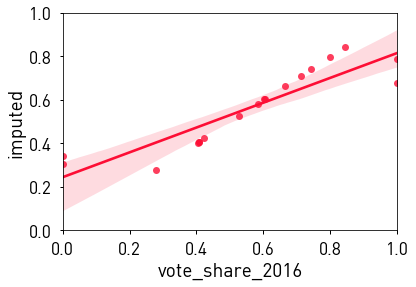With this, we can again compute the efficiency gap:

In :
egap_imputation = dict()
for year in (2014,2016):
chunk = stacked.copy(deep=True)
if year == 2016:
chunk['turnout'] = chunk['turnout_2016']
chunk['vote_share'] = chunk['imputed']
Rwin = chunk.query('vote_share < .5')
Dwin = chunk.query('vote_share >= .5')

Rsurplus = ((1-Rwin.vote_share) -.5) * Rwin.turnout
Rlost = ((1 - Dwin.vote_share)) * Dwin.turnout

Dsurplus = (Dwin.vote_share - .5) * Dwin.turnout
Dlost = (Rwin.vote_share) * Rwin.turnout

Dwaste = Dsurplus.sum() + Dlost.sum()
Rwaste = Rsurplus.sum() + Rlost.sum()

egap_t = (Rwaste - Dwaste) / chunk.turnout.sum()
egap_imputation.update({year:egap_t})
pd.Series(egap_imputation)
Out:
2014   -0.019956
2016   -0.022347
dtype: float64

Here, we see that when we impute the vote shares, the 2016 efficiency gap becomes even more pronounced than when we only consider the contested elections. So, again, deciding on how you want to deal with the uncontested districts is important. This becomes even more important at the state legislative district level, where more districts tend to go uncontested over larger stretches of time. It's worth remembering that Whitford (and many other partisan gerrymandering cases) focus primarily on the statehouse, not Congressional delegations.

Showing uncertainty for empirical measures¶

Given that we can estimate these empirical measures, there's often a question.

How large is too large?

Alternatively,

How is the specific statistic we observe related to the conceptual set of possible statistics?

For empirical measures, there's no explicit distributional theory linking the statistic and its conceptual reference distribution. So, it's fairly common to specify a model for vote shares ($h_i$) & simulate from this model to build up a bank of likely (but not observed) elections. These elections are in the "zone of chance" (Wang's quoting of Scalia there), and reflect outcomes that are plausible: they look like elections that we could have observed. They're generated from the statistical model estimated from elections we did observe, plus some randomness. Later, we'll use this strategy again in the counterfactual measures.

So, this is where much of the finnicky debate (and Robert's' concerns about "sociological gobbldegook") come in. Just like how choosing to impute, retain, or leave out uncontested elections can impact thre statistic significantly, choosing the right model specification can impact analysis significantly. I'll use the strategy I used above for imputation, following from Gelman & King (1994)'s "Unified Method" paper and used extensively in my dissertation.

It requires that you specify a two-cycle model of elections, predicting this year's results using last year's results plus some information about the conditions this year. Alterntaive realizations about this year are simulated from this model, but shrunk towards the observed result as a way of producing more realistic, slightly lower-variance simulations. And, their specification also provides for uncertainty in estimation & potential collinearity between estimands, so that our resulting election simulations are a bit less bland than a standard Gaussian model might produce (e.g. McGann (2016) also considered extensively in my dissertation).

First, we estimate the model¶

$$h_{i,2016} = \alpha h_{i,2014} + \beta_0 + \beta_1 \text{INC} + \epsilon$$

$\text{INC}$ is an ordinal variable governing whether the district had an incumbent Republican ($-1$), an incumbent Democrat ($1$), or no incumbent running ($0$). This isn't really necessary in this application, but I'll return to this exact model in later blogposts. Since we're just working with two years' data, this is effectively a first-order temporal autoregressive model with exogenous components model if you have the time, or an $ARX(1)$ if you don't. But, this is not the case when you include more than two periods of data, which Gelman & King (1994) go into.

Further, epsilon here will be a typical Gaussian white noise term. This is slightly different than what's done in JudgeIt II and what I do in my dissertation, which lets the error variance for each district be proportional to its turnout. This accounts for the fact that we might expect low-turnout election results to be more variable than high turnout ones (or vice versa). But, doing this would complicate the exposition below, so just know that this model can get very high-falutin. All we need it for is to produce the simulated elections in a way that's empirically realistic .

In :
model = smf.ols('imputed ~ vote_share + inc_2016', data=stacked).fit()
In :
model.summary()
/home/ljw/anaconda3/envs/ana/lib/python3.6/site-packages/scipy/stats/stats.py:1334: UserWarning: kurtosistest only valid for n>=20 ... continuing anyway, n=18
"anyway, n=%i" % int(n))
Out:
Dep. Variable: R-squared: imputed 0.986 OLS 0.984 Least Squares 539.7 Wed, 04 Oct 2017 1.06e-14 15:06:25 44.356 18 -82.71 15 -80.04 2 nonrobust
coef std err t P>|t| [0.025 0.975] 0.1346 0.031 4.299 0.001 0.068 0.201 0.7979 0.059 13.535 0.000 0.672 0.924 0.0289 0.011 2.538 0.023 0.005 0.053
 Omnibus: Durbin-Watson: 0.032 1.443 0.984 0.175 -0.081 0.916 2.546 14.7

Now, Gelman & King (1994) uses a special generating function for the counterfactual elections. If you're not comfortable with vector notation, I'm sorry, but it's difficult to avoid here :)

Let's let $\mathbf{h}$ be the observed vote shares for an election in time $t$. Then, let $\mathbf{h}^\circ$ be an artificial election we're trying to construct. Gelman & King (1994) suggests that we draw this from:

$$\mathbf{h}^\circ \sim \mathcal{N}(\alpha \mathbf{h} + (\mathbf{X} - \alpha \mathbf{X}^\circ)\hat{\beta}, (\mathbf{X} - \alpha \mathbf{X}^\circ)\hat{\Sigma}_\beta(\mathbf{X} - \alpha \mathbf{X}^\circ)^T + (1 - \alpha^2)\sigma^2 I)$$

In fact, theirs is a little more complicated (again) since it's pooling over an entire period where the districts stay the same, not a two-cycle period alone. But, for this exposition (again) I'll simplify to avoid some of the nitty-gritty parts (someone's gotta draw the line somewhere). These choices form the morass that any partisan can contest, & rapidly devolves into statistical (not sociological) "gobbledegook."

Proceeding, I'll arrange the information we need:

In :
Sigma = model.cov_params().values
beta_hat = model.params.values.reshape(-1,1)
alpha = beta_hat
X = model.model.exog
Xcirc = model.model.exog #since we're simulating under the same conditions as observed
sigma2 = model.mse_resid
h_obs = model.model.endog.reshape(-1,1)

Construct the relevant quantities:

In :
mean = alpha * h_obs + (X - alpha * Xcirc).dot(beta_hat)
cov = (X - alpha * Xcirc).dot(Sigma).dot((X - alpha * Xcirc).T) + (1 - alpha**2)*sigma2

With these quantities, I'll make 1000 "fake" simulated elections by drawing from a multivariate normal distribution:

In :
fakes = np.random.multivariate_normal(mean.flatten(), cov, size=1000)

To visualize, the red dashed line is the "observed" distribution of district Democratic two-party vote shares and the black shadow around it is the envelope of simulated elections that represent "plausible" but not observed outcomes:

In :
plt.figure(figsize=(2.1*4,4))
plt.title('Simulated Elections, IL 2016')
plt.xlabel("Two-Party Vote %D")
[sns.kdeplot(fake, color='k', alpha=.01) for fake in fakes]
sns.kdeplot(h_obs.flatten(), color='orangered', linestyle='-')
Out:
<matplotlib.axes._subplots.AxesSubplot at 0x7f13ba1df588>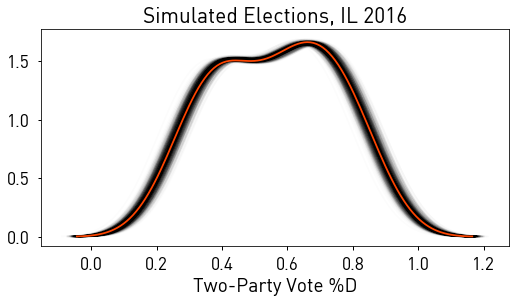Then, like before, we can compute the same statistics about bias.

In :
f = plt.figure(figsize=(2.1*4,4))
ax = plt.gca()
sns.kdeplot(np.median(fakes, axis=1) - fakes.mean(axis=1), label='Simulations', ax=ax)
ax.set_title('Median Minus Mean TPV')
ax.set_xlabel('Two-Party Vote %D')
ax.vlines(np.median(h_obs) - h_obs.mean(), 0, ax.get_ylim() * .25, label='Observed')
ax.legend(frameon=False)
Out:
<matplotlib.legend.Legend at 0x7f13b8ef61d0>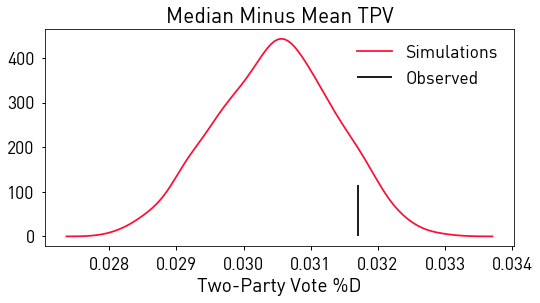Doing the efficiency gap here is a bit flawed, since it requires that we either simulate new turnouts or assume that turnout is nonstochastic. There are some models I've worked with that actually simulate vote share and turnout anew (ahem, Drew Linzer's 2012 paper in Political Analysis, a criminally-underutilized paper), but I won't do that here because it's again too technical to explain Gaussian mixture models in this context.

So, let's just use the varying $\mathbf{h}^\circ$ and compute the efficiency gap holding turnout at observed levels.

In :
egap_simulated = []
turnout = stacked.turnout_2016.values
for fake in fakes:

Dwaste = Dsurplus.sum() + Dlost.sum()
Rwaste = Rsurplus.sum() + Rlost.sum()

egap_i = (Rwaste - Dwaste) / turnout.sum()
egap_simulated.append(egap_i)
egap_simulated = np.asarray(egap_simulated)

With this, the set of simulated efficiency gaps is:

In :
plt.figure(figsize=(2.1*4,4))
sns.kdeplot(egap_simulated, label='simulated')
plt.vlines(-0.022347, 0, plt.gca().get_ylim()*.25, label='observed')
plt.legend(frameon=False)
plt.title('Efficiency Gap')
plt.show()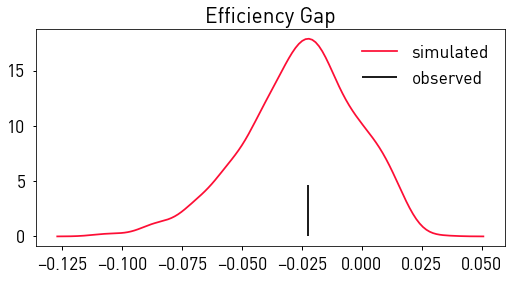Another point of gobbledegook you'll note (akin to what @ElectProject tweets here), there's substantial mass of this simulation distribution beyond zero (i.e. on the far side of zero from the observed value & the mean simulated estimate). If we interpret this simulation distribution as if it were a posterior distribution, its likely that the pseudo-$p$ value on this efficiency gap estimate would indicate negligible bias indistinguishable from zero at a given empirical frequency. This means that claims like:

Only 6 out of 10 simulations show bias towards Republicans, so it's not really "significant."

However, this assumes that the set of elections that we actually observe is sampled by the simulation routine. My dissertation shows that this is probably the case, and using more "realistic" maps of election results (specifically, maps of electoral swing, the change from what's observed and the counterfactual) results in substantively similar conclusions.

Regardless, the steps I've just walked through is often how these measures are deployed:

1. Impute/otherwise handle uncontested elections
2. Compute observed value
3. Construct simulation regime
4. Simulate elections
5. Use simulations to characterize uncertainty

Counterfactual Measures¶

Counterfactual measures use a similar strategy, but measure bias at a specific electoral scenario. For example, the one suggested in Gelman & King (1994) (and endorsed by Grofman & King (2007) after LULAC v. Perry (2006)) is a symmetric bonus standard.

Conceptually, these measures focus on ensuring that a winner's "bonus" is as available to Democrats as it may be to Republicans, and vice versa. This equivalence ensures that the system is "neutral" in the language of Neimi & Deegan (1978), since similarly-situated parties do as well as one another when their winners' bonuses are the same.

So, if Democrats win 60% of the votes & win 70% of the seats, then Republicans should win 70% of the seats when they win 60% of the vote. If they do not, then the system is not neutral, since Republicans either overperform Democrats or underperform Democrats when they win a similar aggregate or average voteshare. Noting the "winner's bonus" of 10% (the percent of seats the Democrats win over and above their vote share), if the winner's bonus is equal for parties, then the system is also neutral.

What's the observed winners bonus in Illinois?¶

In :
print("Dems win {:.2f} % of the two-party vote and had {:.2f}% seats in 2014, a bonus of ~4%".format(
np.average(stacked.vote_share*100, weights=stacked.turnout*100), (stacked.vote_share > .5).mean()*100))
Dems win 51.42 % of the two-party vote and had 55.56% seats in 2014, a bonus of ~4%
In :
print("Dems win {:.2f} % of the two-party vote and had {:.2f}% seats in 2014, a bonus of ~7%".format(
np.average(stacked.vote_share_2016*100, weights=stacked.turnout_2016*100),
(stacked.vote_share_2016 > .5).mean()*100))
Dems win 53.97 % of the two-party vote and had 61.11% seats in 2014, a bonus of ~7%

OK, now how do we construct the hypothetical outcome, when Republicans win what Dems did?¶

Gelman & King (1994) use a simple random effect with mean $\delta$ and variance $(1-\alpha^2)\sigma^2I$ to do this. Thus, the "hypothetical" distribution shown above is actually, in full:

$$\mathbf{h}^\circ \sim \mathcal{N}(\alpha \mathbf{h} + (\mathbf{X} - \alpha \mathbf{X}^\circ)\hat{\beta} + \delta, (\mathbf{X} - \alpha \mathbf{X}^\circ)\hat{\Sigma}_\beta(\mathbf{X} - \alpha \mathbf{X}^\circ)^T + (1 - \alpha^2)\sigma^2 I)$$

Breaking this apart, you can see that it's actually the sum of three parts. One, the observed result (shrunk a bit); two, the uncertainty involved in estimating $\beta$; three, the swing term $\delta$ and its associated variance (which is less than $\sigma^2$): $$\mathbf{h}^\circ = \alpha \mathbf{h} + \mathcal{N}\left( (\mathbf{X} - \alpha \mathbf{X}^\circ)\hat{\beta}, (\mathbf{X} - \alpha \mathbf{X}^\circ)\hat{\Sigma}_\beta(\mathbf{X} - \alpha \mathbf{X}^\circ)^T\right) + \mathcal{N}(\delta, (1 - \alpha^2)\sigma^2 I))$$

where the last term is the electoral swing term. Critically, this assumes that the change in two-party vote share between years (or from one observed outcome to any other scenario) is:

1. spatially homogenous: any place is as likely to swing in any direction as any other place.
2. spatially independent: each place is independent of neighboring places and is just as likely to shift Republican if the neighbor shifts Republican as it is to shift Democrat if the neighbor shifts Republican.
3. spatially homoskedastic: each place is just as volatile as any other place.

These are pretty unrealistic assumptions, IMHO. As an electoral geographer, I was interested in seeing whether or not relaxing these assumptions provides any significant differences in the model. I found mixed results, but it seems that the variance and homogeneity matter a lot more than the dependence. This may be different at different scales of analysis, though, I was only working at US Congressional scales. So, eventhough they're unrealistic, relaxing them did not gain significantly to accuracy in my experience.

Centering the elections¶

So, our simulation above already accounted for a swing variance term, and occurred as if we set $\delta = 0$.

One common measure is to provide an estimate of how many seats the losing party would've won had it done as well as the winner was observed to.

So, to do this, we need to shiff the average vote share of each election up to the average of the observed election. Note here I'm using the average district voteshare rather than the statewide vote share for simplicity, but again, the two are not equivalent. You could do this analysis using the statewide vote share, too, and results may differ.

Unfortunately, each simulation has a slightly different mean voteshare!¶

and they're all distributed near the observed value

In :
plt.figure(figsize=(2.1*4,4))
sns.kdeplot(fakes.mean(axis=1), label='Simulated')
plt.vlines(h_obs.mean(), 0,10, label = 'Observed')
plt.legend(frameon=False)
plt.xlabel("Two-Party Vote, %D")
Out:
<matplotlib.text.Text at 0x7f13b8ee6a58>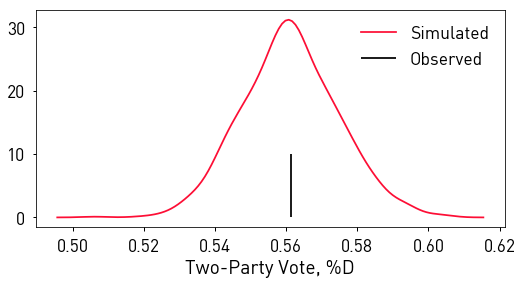So, letting the observed average vote share be $\bar{\mathbf{h}}$, we have to add a different amount to each $\mathbf{h}^\circ$ to ensure its mean, $\bar{\mathbf{h}^\circ}$, is equal to $1 - \bar{\mathbf{h}}$, what Republicans won in the observed conditions. This is obtainable from some algebra. You use the difference between the observed and simulated means to center each simulation on the observed mean.

Then, you "flip" these by subtracting them from one to get the "tables turned" scenario, simulated elections where Democrats did as well as Republicans were observed to have done.

This would amount to adding a uniquely-meaned random effect $\delta$ to each election forcing it to be constrained to have the correct mean. An alternative I'll use here is to ensure that only the batch mean is centered on 50%. This means that some simulations will be slightly above .5 and some slightly below .5, but the batch as a whole will estimate near the median. This is accomplished by specifying a single mean.

In :
constrained = (fakes + (h_obs.mean() - fakes.mean(axis=1).mean()))
tables_turned = (fakes + ((1 - h_obs.mean()) - fakes.mean(axis=1).mean()))
In :
plt.figure(figsize=(2.1*4,4))
plt.title('Tables-Turned Counterfactual')
[sns.kdeplot(turn, color='k', alpha=.01) for turn in tables_turned]
sns.kdeplot((1 - h_obs).flatten(), color='orangered', label='Observed')
plt.vlines(tables_turned.mean(axis=1), 0,.1, color='b', label="CF mean")
plt.xlabel("Two-Party Vote, %D")
plt.legend(frameon=False, loc='upper right')
plt.show()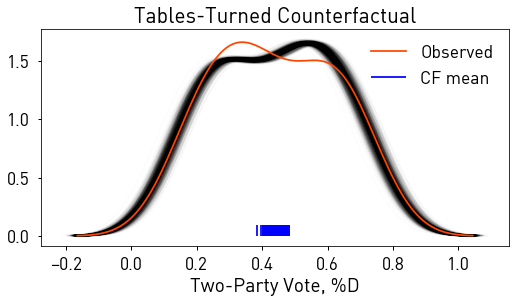Now, the "seat bonus" measure is the difference between the expected seat share ($\bar{s}$) won at the observed vote share and the seats won by the opponent when tables are turned:

$$B = E[\bar{s} \ \vert \ \bar{\mathbf{h}}] - \left(1 - E[\bar{s} \ \vert \ 1 - \bar{\mathbf{h}}]\right)$$

with our constrained and tables_turned simulations, we can easily compute this:

In :
dem_share_at_observed = (constrained >= .5).mean(axis=1).mean()
dem_share_at_tables_turned = (1 - (tables_turned >= .5).mean(axis=1).mean())
extra_bonus_at_observed = dem_share_at_observed - dem_share_at_tables_turned
In :
print("Seat share bonus at observed voteshare: {:.4f}% ({:.1f} seats)".format(
extra_bonus_at_observed*100, extra_bonus_at_observed * 18))
Seat share bonus at observed voteshare: 0.4611% (0.1 seats)

So, Dems win effectively the same number of seats at 53% vote share as Republicans would be projected to win if they were to win an average district voteshare of 53%, given what we have observed about the elections. Since you can't really win a tenth of a seat, this difference in bonus is so small that it's probably not felt.

Median Bonus¶

Another measure involves the evaluation of simulations exactly at $\bar{\mathbf{h}^\circ} = .5$. In this case, since both parties recieve the same average vote share, this provides a pretty direct measure of the anticipated bias:

$$B_{.5} = 2E[\bar{s} \ | \ \bar{\mathbf{h}} = .5] - 1$$

Further, it's always "closer" to the observed result to use this simulation than it is to use a tables-turned scenario, so this measure is often preferred to the tables turned method.

In :
median_constrained = fakes + (.5 - fakes.mean(axis=1).mean())
In :
[sns.kdeplot(const, color='k', alpha=.01) for const in median_constrained]
plt.vlines(median_constrained.mean(axis=1), 0,.1,color='b', label='CF Mean')
plt.title('Simulations At Median')
plt.xlabel('Two-Party Vote %D')
plt.show()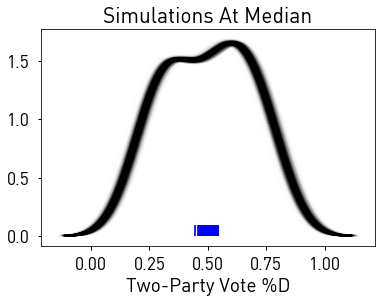Using the median estimate of the difference in seat bonus:

In :
Es_at_median = (median_constrained > .5).mean(axis=1).mean()
Bmedian = Es_at_median * 2 - 1
In :
print("Seat share bonus at observed voteshare: {:.4f}% ({:.1f} seats)".format(
Bmedian*100, Bmedian * 18))
Seat share bonus at observed voteshare: 10.5000% (1.9 seats)

So, at median, the bonus is around 10% of the delegation, or $.1 \times 18 \approx 2$ seats in the Illinois congressional delegation. This is an order of magnitude more seats than that at the observed vote shrae.

McGhee (2014) highlights how these counterfactual measures can be inconsistent depending on the range over which they are evaluated when arguing for the efficiency gap. Some (e.g. JudgeIt II) recommend taking an average of the values computed in a given range. This seems reasonable to me, in addition to using the point estimate at median. But, again, the sensitivity to the estimate and precise choice of ranges is "gobbledegook" that might be optimized by partisan actors.

Showing uncertainty for counterfactual bias measures¶

Just like empirical measures, you can use replications to generate a simulation distribution for the uncertainty measures. The process is identical to computing them once, however. You simply generate a brand new set of simulations, compute the expected bias, and repeat.

Alternatively, if you want to be sparing of your CPU cycles, you can summarize the raw bias estimator without computing the expectations of each "batch."

Since you probably understand that just repeating the above process for a new call to np.random.multivariate_normal is easily done in a standard for-loop, I'll show you what I mean by using the raw estimator below.

Taking the observed bias measure first, note that most of our calls to .mean had the following:

X.mean(axis=1).mean()

This happens because we're taking the mean over simulations, axis=1, and then taking the average over all of those averages to construct an expected value over simulation.

If we just avoid the last mean() call until the very end, we can "recycle" the set of estimators:

In :
dem_shares_at_observed = (constrained >= .5).mean(axis=1)
dem_shares_at_tables_turned = (1 - (tables_turned >= .5).mean(axis=1))
extra_bonuses_at_observed = dem_shares_at_observed - dem_shares_at_tables_turned
In :
plt.hist(extra_bonuses_at_observed)
values,counts = np.unique(extra_bonuses_at_observed, return_counts=True)
pd.DataFrame(np.vstack((values,counts)).T, columns=['Values', 'Count'])
Out:
Values Count
0 -0.111111 1.0
1 -0.055556 63.0
2 0.000000 857.0
3 0.055556 13.0
4 0.111111 63.0
5 0.166667 3.0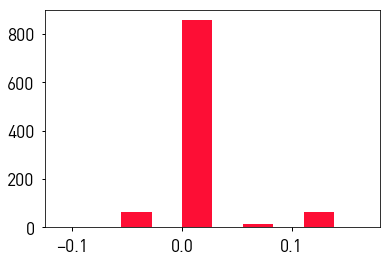When we take the average of these, we still get the same estimate when we didn't use the internal means:

In :
np.testing.assert_allclose(extra_bonuses_at_observed.mean(), extra_bonus_at_observed) #equivalent to float tolerance

But, we also get a distribution of the possible seat share differences. Since seat shares are integral, in that there are only a finite number of possible seat shares, this the simulation frequency distribution for the statistic can be summarized in a more appropriate way than just using the mean to smooth out the estimate.

This also works for the bias at median:

In :
median_constrained = fakes + (.5 - fakes.mean(axis=1).mean())
In :
Bmedians = (2*(median_constrained > .5).mean(axis=1) - 1)
In :
plt.hist(Bmedians)
values,counts = np.unique(Bmedians, return_counts=True)
pd.DataFrame(np.vstack((values,counts)).T, columns=['Values', 'Count'])
Out:
Values Count
0 -0.222222 1.0
1 -0.111111 1.0
2 0.000000 53.0
3 0.111111 942.0
4 0.222222 3.0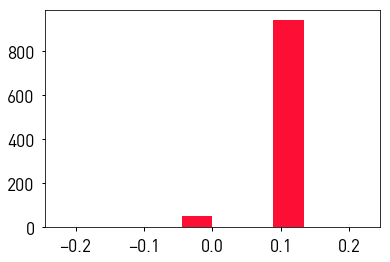In :
np.testing.assert_allclose(Bmedians.mean(), Bmedian) #equivalent to float tolerance

Conclusion¶

So, I hope this provides a little bit of a quantitative intro to how these kinds of measures are generated, and where the gobbledegook might seep in. The efficiency gap, while cool, new, and shiny, is no exception to the gobbledegook that's been floating about in this field ever since the first "modern" studies of strucual election modeling in the 1950s (Maurice Kendall's exploration of the Cube Rule). A few pressure points that are helpful to keep yourself honest.

1. How does the anlaysis treat uncontested elections?
2. Does the result provide any indication of how uncertain the estimate is with respect to the observed election/available elections under the plan?
3. Does the specification of the model have reasonable fit & generate elections that look like the elections we observe both spatially and distributionally?
4. Is any attempt made to control for confounding factors? I'll follow up with this in another blogpost.

Saying

only now, armed with numbers, can we defeat the gerrymander!

is a gross misunderstanding of the state of play. While the efficiency gap is new, it's not the first bias number... nowhere close. Further, saying that the efficiency gap is somehow simpler, more intuitive, or empirically more sound misrepresents ongoing debate about which measures are appropriate and valid in the literature & in practitioner's own minds. For a (dis)heartening reminder, my dissertation included interviews with individuals who sit on nonpartisan/independent redistricting commissions in a few states & none interviewed recalled using these explicitly in their reasoning about plans. So, take all of these numbers with a grain of salt and my colleagues and I will keep working on making better ones.

Lastly, I'd encourage many people to avoid thinking about things in terms of the efficiency gap or bust. It's really not that simple. This is fundamentally a statistical problem; to think we've suddenly solved it because a new estimator reprises & revamps arguments made by R.H. Brookes in 1960s New Zealand is silly. That said, I hope you can acknowledge that scientifically-grounded skepticism is not necessarily skepticicsm of scientific progress, and approach these things with an understanding that it's not efficiency gap or bust.